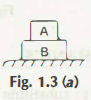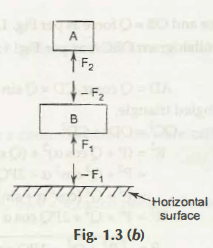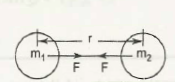Mechanics is based on the different laws and with the help of these laws any proper decision is taken. What are these laws? These are as follows –

• Newton’s First Law Of Motion –

This law states that a body at rest remains state of rest or a body in motion remains state of a uniform motion unless or until any outer force acts on it. This is also known as the Law of Inertia. A lot of examples can be seen in the daily life of people as people go backward when the train starts at a sudden.• Newton’s second law of Motion  –

In this statement Newton stated that the acceleration of a moving body or an object is directly proportional to the value or the magnitude of the force in the same direction of the force and is inversely  proportional to the mass in the body. It means it is difficult to move a fully loaded truck than an empty truck.• Newton’s Third Law of Motion –

It explains that each and every action there is an equal and opposite reaction.Law Of Gravitation or Gravitaional Attraction

According to the Law of Gravitational Attraction the two bodies attract each other with a great force and thus the magnitude of the force is directly proportional to the product of their masses and inversely proportion to the square of the distance between them.

It means F ∝ m1.m2/r2

F= G . m1 m2/r2

Here, G is a Gravitational Constant.

These laws have a great significance because this explains that how movement of different planets take place in the solar system. Newton finds out only to understand the attraction between the sun and the different planets. However, this law is beneficial in the field of Mechanics in various ways. The attraction of the bodies increases with a decrease in the distance between the bodies.

Links of Next Mechanical Engineering Topics:-### Customer Reviews

My Homework Help
Rated 5.0 out of 5 based on 510 customer reviews at